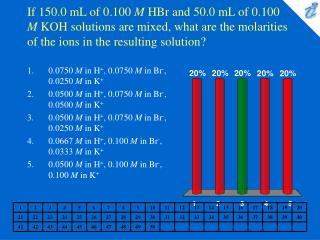DownloadDownload PresentationIf 150.0 mL of 0.100 M HBr and 50.0 mL of 0.100 M KOH solutions are mixed, what are the molarities of the ions in th

# If 150.0 mL of 0.100 M HBr and 50.0 mL of 0.100 M KOH solutions are mixed, what are the molarities of the ions in th

Télécharger la présentation## If 150.0 mL of 0.100 M HBr and 50.0 mL of 0.100 M KOH solutions are mixed, what are the molarities of the ions in th

- - - - - - - - - - - - - - - - - - - - - - - - - - - E N D - - - - - - - - - - - - - - - - - - - - - - - - - - -
##### Presentation Transcript

1. If 150.0 mL of 0.100 M HBr and 50.0 mL of 0.100 M KOH solutions are mixed, what are the molarities of the ions in the resulting solution? • 0.0750 M in H+, 0.0750 M in Br-, 0.0250 M in K+ • 0.0500 M in H+, 0.0750 M in Br-, 0.0500 M in K+ • 0.0500 M in H+, 0.0750 M in Br-, 0.0250 M in K+ • 0.0667 M in H+, 0.100 M in Br-, 0.0333 M in K+ • 0.0500 M in H+, 0.100 M in Br-, 0.100 M in K+

2. A 0.742-gram sample of KHP, KC6H4(COO)(COOH), reacts with 35.0 mL of Ba(OH)2 solution. What is the molarity of the Ba(OH)2 solution? • 0.127 M • 0.0636 M • 0.0520 M • 0.208 M • 0.104 M

3. Calculate the normality of a solution that contains 4.5 g of (COOH)2 in 3000. mL of solution. (Assume the (COOH)2 is to be completely neutralized.) • 0.033 N • 0.045 N • 0.066 N • 0.090 N • 0.12 N

4. A solution of H2SO4 contains 9.11 mg of H2SO4 per mL. What is the normality of the solution? (Assume the H2SO4 is to be completely neutralized by a base.) • 0.0482 N • 0.684 N • 0.186 N • 0.372 N • 0.0930 N

5. How many equivalent weights of phosphoric acid are contained in 500. mL of 2.00 M phosphoric acid? (Assume the acid is to be completely neutralized by a base.) • 1.00 eq • 2.00 eq • 3.00 eq • 4.00 eq • 6.00 eq

6. What mass of NaOH would be required to neutralize the acid in 75.0 mL of 0.880 N HCl? • 2.64 g • 5.28 g • 2.6 kg • 3.83 g • 6.60 g

7. If 20.8 mL of Ba(OH)2solution reacts with 0.306 g of KHP, KC6H4(COO)(COOH), what is the normality of the Ba(OH)2 solution? • 0.0361 N • 0.144 N • 0.300 N • 0.175 N • 0.0721 N

8. What is the oxidation number of phosphorus in KH2PO4? • +1 • +2 • +3 • +4 • +5

9. What is the oxidation number of P in the H2PO4-ion? • -3 • +2 • +3 • +4 • +5

10. Balance the following formula unit equation for a reaction. What is the sum of all of the coefficients? K2Cr2O7 + HCl {image} KCl + CrCl3 + Cl2+ H2O • 20 • 22 • 26 • 29 • 32

11. Balance the following net ionic equation. What is the sum of the coefficients? (COOH)2 + MnO4- + H+ {image} CO2 + Mn2++ H2O • 27 • 29 • 31 • 33 • 35

12. Balance the following net ionic equation. What is the sum of the coefficients? MnO4- + Se2- {image} MnO2+ Se (basic solution) • 20 • 22 • 24 • 26 • 28

13. An impure 0.500-gram sample of FeSO4 reacts with 20.0 mL of 0.0200 M KMnO4. Assuming that the impurities do not react with KMnO4, what is the percentage of FeSO4 in the sample? MnO4- + 8H+ + 5Fe2+ {image} Mn2+ + 5Fe3+ + 4H2O • 52.3% • 56.6% • 60.8% • 64.5% • 69.2%

14. A solution of Fe(NH4)2(SO4)2 · 6H2O containing 2.10 grams of the compound is titrated with acidic Na2Cr2O7 solution. If 41.6 mL of the Na2Cr2O7 solution is required, what is the molarity of the Na2Cr2O7 solution? The reaction products include Cr3+ and Fe3+. • 0.129 M • 0.0215 M • 0.0644 M • 0.0387 M • 0.0744 M